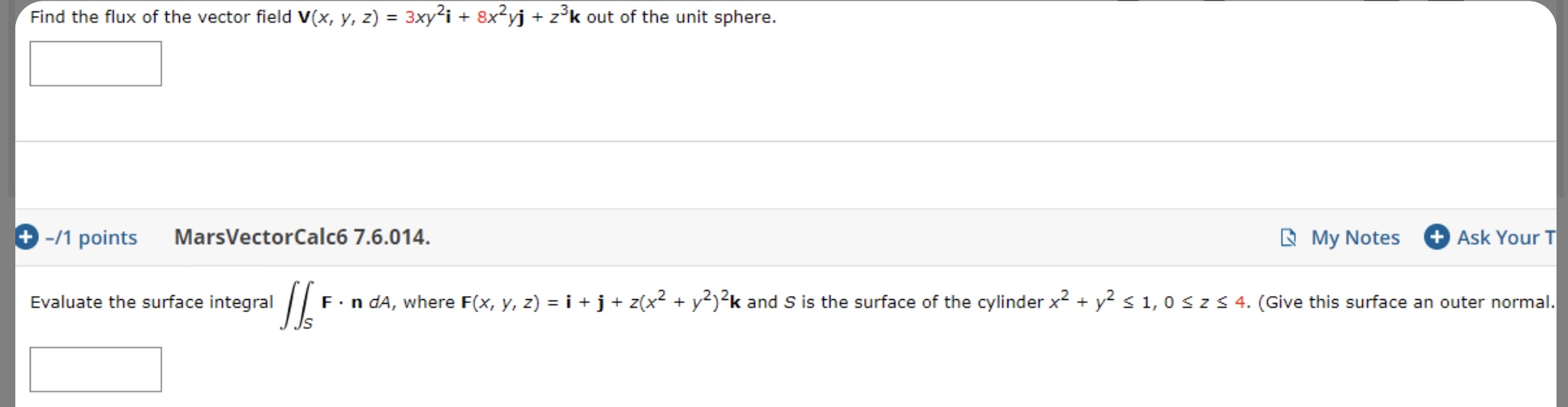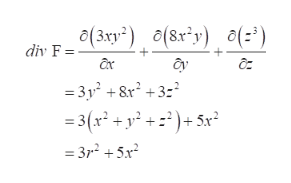# Find the flux of the vector field V(x, y, z) 3xyi 8x2yj zk out of the unit sphere.MarsVectorCalc6 7.6.014+-/1 pointsAsk Your TMy NotesEvaluate the surface integralF n dA, where F(x, y, z) i +j + z(x2 + y)2k and S is the surface of the cylinder x+ y s 1, 0 S z4. (Give this surface an outer normal.

Question
106 viewshelp_outlineImage TranscriptioncloseFind the flux of the vector field V(x, y, z) 3xyi 8x2yj zk out of the unit sphere. MarsVectorCalc6 7.6.014 +-/1 points Ask Your T My Notes Evaluate the surface integral F n dA, where F(x, y, z) i +j + z(x2 + y)2k and S is the surface of the cylinder x+ y s 1, 0 S z 4. (Give this surface an outer normal. fullscreen
check_circle

Step 1

The flux through the surface S and solid region E of a vector field F is calculated by,

Step 2

Here V(x,y,z) is given by,

Step 3

Find div F for the divergence theorem and substitute, x2+...help_outlineImage Transcriptioncloseo(3xy2) (8ry) o(-) div F =- =3y +8r2 +3 3(x +y? +z)+ 5x* = 3r2 5 fullscreen

### Want to see the full answer?

See Solution

#### Want to see this answer and more?

Solutions are written by subject experts who are available 24/7. Questions are typically answered within 1 hour.*

See Solution
*Response times may vary by subject and question.
Tagged in

### Math